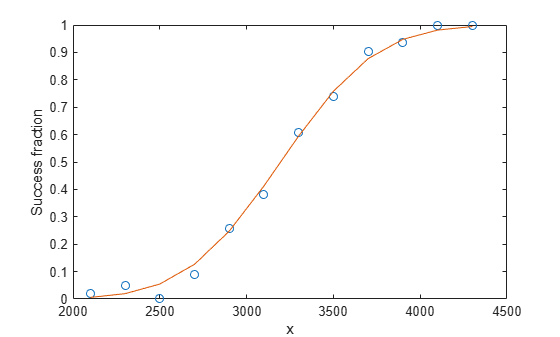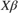# glmval

Generalized linear model values

## Syntax

yhat = glmval(b,X,link) [yhat,dylo,dyhi] = glmval(b,X,link,stats) [...] = glmval(...,param1,val1,param2,val2,...) 

## Description

yhat = glmval(b,X,link) computes predicted values for the generalized linear model with link function link and predictors X. Distinct predictor variables should appear in different columns of X. b is a vector of coefficient estimates as returned by the glmfit function. link can be any of the character vectors, string scalars, or custom-defined link functions used as values for the 'link' name-value pair argument in the glmfit function.

Note

By default, glmval adds a first column of 1s to X, corresponding to a constant term in the model. Do not enter a column of 1s directly into X. You can change the default behavior of glmval using the 'constant' parameter.

[yhat,dylo,dyhi] = glmval(b,X,link,stats) also computes 95% confidence bounds for the predicted values. When the stats structure output of the glmfit function is specified, dylo and dyhi are also returned. dylo and dyhi define a lower confidence bound of yhat-dylo, and an upper confidence bound of yhat+dyhi. Confidence bounds are nonsimultaneous, and apply to the fitted curve, not to a new observation.

[...] = glmval(...,param1,val1,param2,val2,...) specifies optional parameter name/value pairs to control the predicted values. Acceptable parameters are listed in this table:

ParameterValue

'confidence' — the confidence level for the confidence bounds

A scalar between 0 and 1

'size' — the size parameter (N) for a binomial model

A scalar, or a vector with one value for each row of X

'offset' — used as an additional predictor variable, but with a coefficient value fixed at 1.0

A vector

'constant'
• 'on' — Includes a constant term in the model. The coefficient of the constant term is the first element of b.

• 'off' — Omit the constant term

'simultaneous' — Compute simultaneous confidence intervals (true), or compute non-simultaneous confidence intervals (default false)true or false

## Examples

collapse all

Fit a generalized linear regression model, and compute predicted (estimated) values for the predictor data using the fitted model.

Create a sample data set.

x = [2100 2300 2500 2700 2900 3100 ... 3300 3500 3700 3900 4100 4300]'; n = [48 42 31 34 31 21 23 23 21 16 17 21]'; y = [1 2 0 3 8 8 14 17 19 15 17 21]';

x contains the predictor variable values. Each y value is the number of successes in the corresponding number of trials in n.

Fit a probit regression model for y on x.

b = glmfit(x,[y n],'binomial','Link','probit');

Compute the estimated number of successes.

yfit = glmval(b,x,'probit','Size',n);

Plot the observed success percent and estimated success percent versus the x values.

plot(x,y./n,'o',x,yfit./n,'-')Enter sample data.

x = [2100 2300 2500 2700 2900 3100 ... 3300 3500 3700 3900 4100 4300]'; n = [48 42 31 34 31 21 23 23 21 16 17 21]'; y = [1 2 0 3 8 8 14 17 19 15 17 21]';

Each y value is the number of successes in corresponding number of trials in n, and x contains the predictor variable values.

Define three function handles, created by using @, that define the link, the derivative of the link, and the inverse link for a probit link function,. Store the handles in a cell array.

link = @(mu) norminv(mu); derlink = @(mu) 1 ./ normpdf(norminv(mu)); invlink = @(resp) normcdf(resp); F = {link, derlink, invlink};

Fit a generalized linear model for y on x by using the link function that you defined.

b = glmfit(x,[y n],'binomial','link',F);

Compute the estimated number of successes. Plot the observed and estimated percent success versus the x values.

yfit = glmval(b,x,F,'size',n); plot(x, y./n,'o',x,yfit./n,'-','LineWidth',2)Train a generalized linear model, and then generate code from a function that classifies new observations based on the model. This example is based on the Use Custom-Defined Link Function example.

Enter the sample data.

x = [2100 2300 2500 2700 2900 3100 ... 3300 3500 3700 3900 4100 4300]'; n = [48 42 31 34 31 21 23 23 21 16 17 21]'; y = [1 2 0 3 8 8 14 17 19 15 17 21]'; 

Suppose that the inverse normal pdf is an appropriate link function for the problem.

Define a function named myInvNorm.m that accepts values ofand returns corresponding values of the inverse of the standard normal cdf.

function in = myInvNorm(mu) %#codegen %myInvNorm Inverse of standard normal cdf for code generation % myInvNorm is a GLM link function that accepts a numeric vector mu, and % returns in, which is a numeric vector of corresponding values of the % inverse of the standard normal cdf. % in = norminv(mu); end 

Define another function named myDInvNorm.m that accepts values ofand returns corresponding values of the derivative of the link function.

function din = myDInvNorm(mu) %#codegen %myDInvNorm Derivative of inverse of standard normal cdf for code %generation % myDInvNorm corresponds to the derivative of the GLM link function % myInvNorm. myDInvNorm accepts a numeric vector mu, and returns din, % which is a numeric vector of corresponding derivatives of the inverse % of the standard normal cdf. % din = 1./normpdf(norminv(mu)); end 

Define another function named myInvInvNorm.m that accepts values ofand returns corresponding values of the inverse of the link function.

function iin = myInvInvNorm(mu) %#codegen %myInvInvNorm Standard normal cdf for code generation % myInvInvNorm is the inverse of the GLM link function myInvNorm. % myInvInvNorm accepts a numeric vector mu, and returns iin, which is a % numeric vector of corresponding values of the standard normal cdf. % iin = normcdf(mu); end 

Create a structure array that specifies each of the link functions. Specifically, the structure array contains fields named 'Link', 'Derivative', and 'Inverse'. The corresponding values are the names of the functions.

link = struct('Link','myInvNorm','Derivative','myDInvNorm',... 'Inverse','myInvInvNorm') 
link = struct with fields: Link: 'myInvNorm' Derivative: 'myDInvNorm' Inverse: 'myInvInvNorm' 

Fit a GLM for y on x using the link function link. Return the structure array of statistics.

[b,~,stats] = glmfit(x,[y n],'binomial','link',link); 

b is a 2-by-1 vector of regression coefficients.

In your current working folder, define a function called classifyGLM.m that:

• Accepts measurements with columns corresponding to those in x, regression coefficients whose dimensions correspond to b, a link function, the structure of GLM statistics, and any valid glmval name-value pair argument

• Returns predictions and confidence interval margins of error

function [yhat,lo,hi] = classifyGLM(b,x,link,varargin) %#codegen %CLASSIFYGLM Classify measurements using GLM model % CLASSIFYGLM classifies the n observations in the n-by-1 vector x using % the GLM model with regression coefficients b and link function link, % and then returns the n-by-1 vector of predicted values in yhat. % CLASSIFYGLM also returns margins of error for the predictions using % additional information in the GLM statistics structure stats. narginchk(3,Inf); if(isstruct(varargin{1})) stats = varargin{1}; [yhat,lo,hi] = glmval(b,x,link,stats,varargin{2:end}); else yhat = glmval(b,x,link,varargin{:}); end end 

Generate a MEX function from classifyGLM.m. Because C uses static typing, codegen must determine the properties of all variables in MATLAB® files at compile time. To ensure that the MEX function can use the same inputs, use the -args argument to specify the following in the order given:

• Regression coefficients b as a compile-time constant

• In-sample observations x

• Link function as a compile-time constant

• Resulting GLM statistics as a compile-time constant

• Name 'Confidence' as a compile-time constant

• Confidence level 0.9

To designate arguments as compile-time constants, use coder.Constant.

codegen -config:mex classifyGLM -args {coder.Constant(b),x,coder.Constant(link),coder.Constant(stats),coder.Constant('Confidence'),0.9} 
Code generation successful. 

codegen generates the MEX file classifyGLM_mex.mexw64 in your current folder. The file extension depends on your system platform.

Compare predictions by using glmval and classifyGLM_mex. Specify name-value pair arguments in the same order as in the -args argument in the call to codegen.

[yhat1,melo1,mehi1] = glmval(b,x,link,stats,'Confidence',0.9); [yhat2,melo2,mehi2] = classifyGLM_mex(b,x,link,stats,'Confidence',0.9); comp1 = (yhat1 - yhat2)'*(yhat1 - yhat2); agree1 = comp1 < eps comp2 = (melo1 - melo2)'*(melo1 - melo2); agree2 = comp2 < eps comp3 = (mehi1 - mehi2)'*(mehi1 - mehi2); agree3 = comp3 < eps 
agree1 = logical 1 agree2 = logical 1 agree3 = logical 1 

The generated MEX function produces the same predictions as predict.

## References

 Dobson, A. J. An Introduction to Generalized Linear Models. New York: Chapman & Hall, 1990.

 McCullagh, P., and J. A. Nelder. Generalized Linear Models. New York: Chapman & Hall, 1990.

 Collett, D. Modeling Binary Data. New York: Chapman & Hall, 2002.

## Version History

Introduced before R2006a